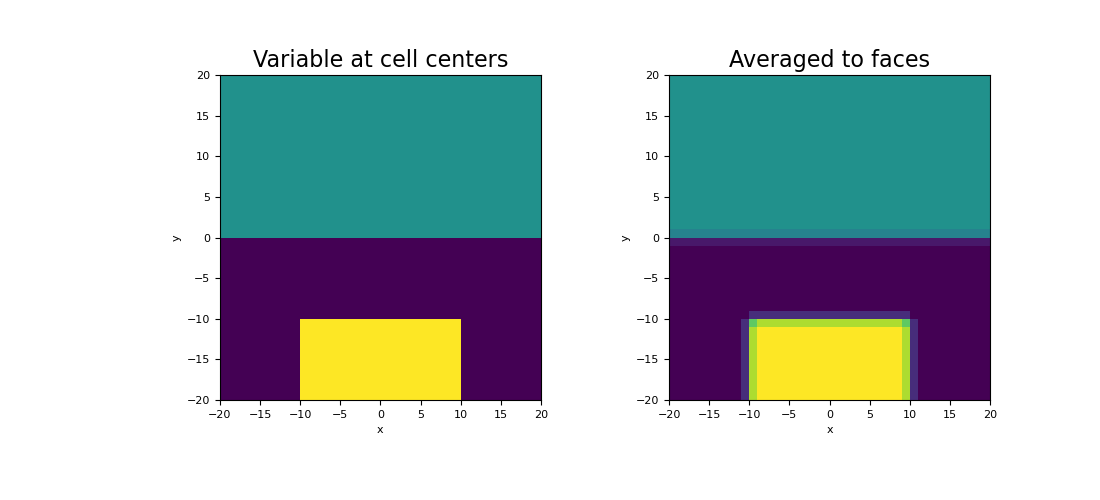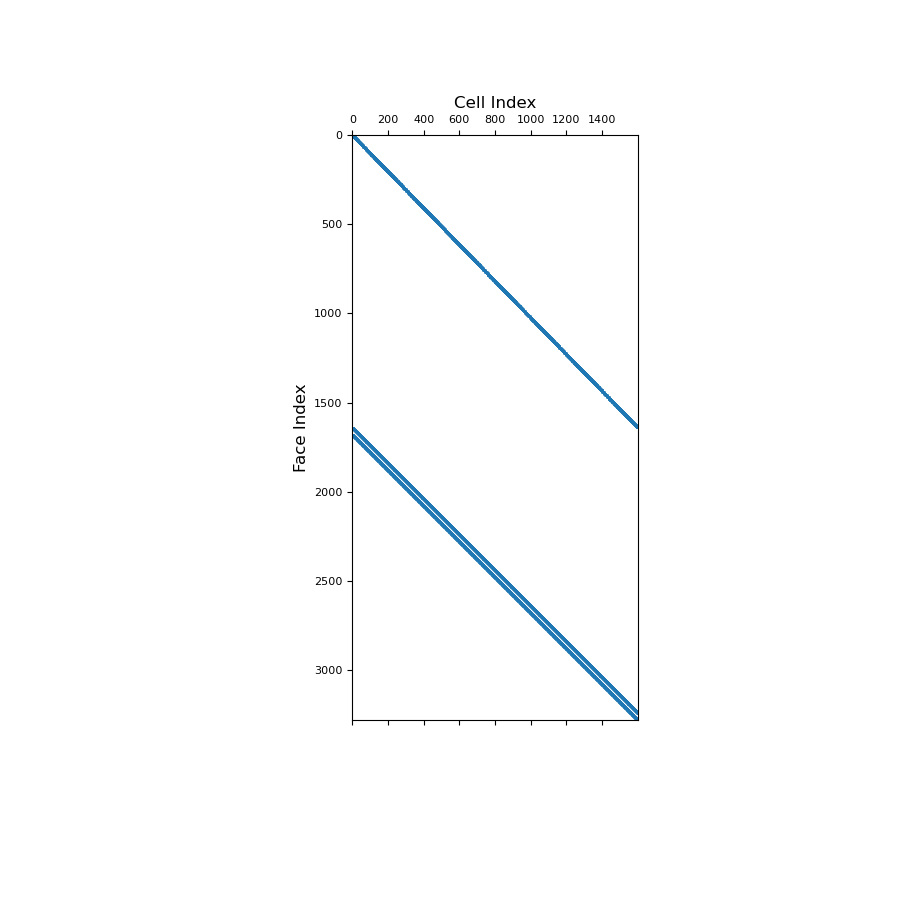# discretize.TensorMesh.average_cell_to_face¶

property TensorMesh.average_cell_to_face

Averaging operator from cell centers to faces (scalar quantities).

This property constructs an averaging operator that maps scalar quantities from cell centers to face. This averaging operator is used when a discrete scalar quantity defined cell centers must be projected to faces. Once constructed, the operator is stored permanently as a property of the mesh. See notes.

Returns
(n_faces, n_cells) scipy.sparse.csr_matrix

The scalar averaging operator from cell centers to faces

Notes

Let $$\boldsymbol{\phi_c}$$ be a discrete scalar quantity that lives at cell centers. average_cell_to_face constructs a discrete linear operator $$\mathbf{A_{cf}}$$ that projects $$\boldsymbol{\phi_c}$$ to faces, i.e.:

$\boldsymbol{\phi_f} = \mathbf{A_{cf}} \, \boldsymbol{\phi_c}$

where $$\boldsymbol{\phi_f}$$ approximates the value of the scalar quantity at the faces. For each face, we are performing a weighted average between the values at adjacent cell centers. In 1D, where adjacent cells $$i$$ and $$i+1$$ have widths $$h_i$$ and $$h_{i+1}$$, $$\phi$$ on face is approximated by:

$\phi_{i \! + \! 1/2} \approx \frac{h_{i+1} \phi_i + h_i \phi_{i+1}}{h_i + h_{i+1}}$

On boundary faces, nearest neighbour is used to extrapolate the value from the nearest cell center. Once the operator is construct, the averaging is implemented as a matrix vector product, i.e.:

phi_f = Acf @ phi_c


Examples

Here we compute the values of a scalar function at cell centers. We then create an averaging operator to approximate the function on the faces. We choose to define a scalar function that is strongly discontinuous in some places to demonstrate how the averaging operator will smooth out discontinuities.

We start by importing the necessary packages and defining a mesh.

>>> from discretize import TensorMesh
>>> import numpy as np
>>> import matplotlib.pyplot as plt

>>> h = np.ones(40)
>>> mesh = TensorMesh([h, h], x0="CC")


Create a scalar variable at cell centers

>>> phi_c = np.zeros(mesh.nC)
>>> xy = mesh.cell_centers
>>> phi_c[(xy[:, 1] > 0)] = 25.0
>>> phi_c[(xy[:, 1] < -10.0) & (xy[:, 0] > -10.0) & (xy[:, 0] < 10.0)] = 50.0


Next, we construct the averaging operator and apply it to the discrete scalar quantity to approximate the value at the faces.

>>> Acf = mesh.average_cell_to_face
>>> phi_f = Acf @ phi_c


Plot the results

Expand to show scripting for plot
>>> fig = plt.figure(figsize=(11, 5))
>>> mesh.plot_image(phi_c, ax=ax1, v_type="CC")
>>> ax1.set_title("Variable at cell centers", fontsize=16)
>>> mesh.plot_image(phi_f, ax=ax2, v_type="F")
>>> ax2.set_title("Averaged to faces", fontsize=16)
>>> plt.show()Below, we show a spy plot illustrating the sparsity and mapping of the operator.

Expand to show scripting for plot
>>> fig = plt.figure(figsize=(9, 9))